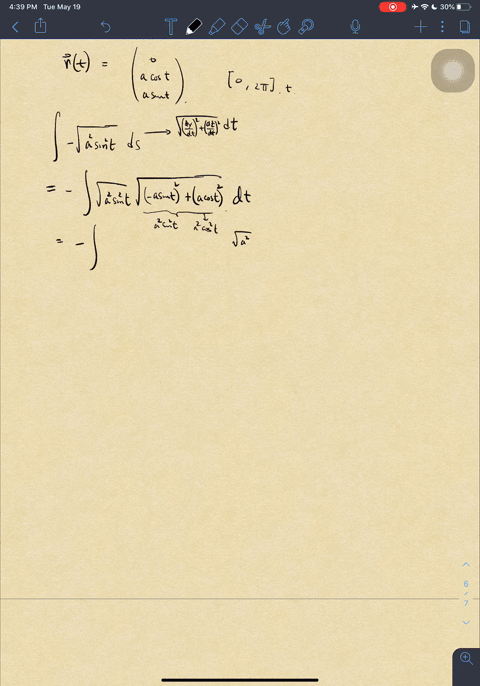🎉 The Study-to-Win Winning Ticket number has been announced! Go to your Tickets dashboard to see if you won! 🎉View Winning Ticket### Integrate $f(x, y, z)=-\sqrt{x^{2}+z^{2}}$ over t…

08:46University of California, Berkeley
Problem 17

# Integrate $f(x, y, z)=(x+y+z) /\left(x^{2}+y^{2}+z^{2}\right)$ over the path $\mathbf{r}(t)=t \mathbf{i}+t \mathbf{j}+t \mathbf{k}, 0<a \leq t \leq b$.

## Discussion

You must be signed in to discuss.

## Video Transcript

Okay, folks. So now we're gonna take a look at this problem. Problem number 17 on your book. Um, this is a line growth. We have this function app, which is a function of position. Let me write down the function here. Um X plus y plus z divided by X squared plus y squared plus eggs eastward. Okay, so we had this function here, and we would like to integrate over, integrate over the path which is given by our parameter prized by the variable t on. I'm gonna write it this way. We have tea for X and T for J and T for for K X, Y and Z are both functions of tea, and they're both the same function. They're both t um and we're gonna t is going to range from from a all the way to be. Um, so this is a very simple problem. Um, we're just going to write it this way. We're gonna you integrate along the curve, uh, of f multiplied by DS. That's the That's the earth. That's the idea. Um, along the curve, because F is a function of x, y and Z, but X, y and Z here all functions of tea. So let me rewrite the function as a function of teeth So X as a function of tea is just tea. Same as why Samos Z so f can be written in a very simple way. So for the nominator we have t squared plus t squared plus t spurred. Okay, Multiplied by DS if you remember what DS is, the s is the infinite asthma line segment which I could write with respect to t as the square root of the three. I guess you can call it velocities. Some people you can you can kind of visualize as a velocity because it's just, um the exit e plus do I d t pleasant Easy, DT. But you know, it's just rid of change of X, y and Z with respect to the variable t. So you can kind of visualize as a philosophy. That's how I visualize it. X is a function of desires TV but so DT So the derivative of tea with your subjectivity is just one. So one squared is still one same as why, same as easy. They're all the same function and you take the derivative of the function with respect to you. Get one. All right. So, uh, and don't forget the integration limits, which is between a and be That's ah, that's really the basic idea we have done. The way we have done, like, 80% of the problem there are. All we have to do now is to simplify it and see what we get. So we have square root of three, which I'm gonna pull out of the integral because that's a constant. That's a constant. Um, we have for the denominator, we have three d. I mean, for the new murder, we have three t over three t squared DT. Okay, so, you know, we're gonna have ah, route three one over t b t. Which I'm going to rewrite as t of to the power of to the power of negative one. You feed. You didn't grow on that. You get natural log. Right? Um, natural log of tea. Um, from a to be No, we're done with this video. Basically natural. Log of ah, be over a notice here. I I kind of jumped the stuff here at the step it I could have done Is this square root of three of natural August B minus natural log of a. But this I just simplified it into this. They're the same thing. It's one of the properties of logarithms, All right, I think that's it for this video. This right here is our finally answer for this problem from number 17. And we're done. No, this year that the A is is actually strictly bigger than zero. That's that's why they they explicitly told you that a has to be bigger than zero. Because if it's not, we're running into a problem here because we have a on the denominator here and we cannot divide by zero. So a must be strictly bigger than zero. All right, that's it for this video. Thank you very much in boy.